﻿ 基于云分段最优熵算法的航行异常轨迹识别研究
 舰船科学技术2022, Vol. 44Issue (7): 150-153    DOI: 10.3404/j.issn.1672-7649.2022.07.030PDF

Research on navigation anomaly trajectory recognition based on cloud segmented optimal entropy algorithm
CHEN Xiao-hai, GAN DU-fen
The Computer Engineering College, Guilin University of Electronic Technology, Beihai 536000, China
Abstract: The rapid development of marine transportation industry puts forward higher requirements for the ship management level of large shipping companies. With the rapid increase of the number of ships, the identification and monitoring technology of ship navigation abnormal trajectory has become a research hotspot. This paper studies how to improve the recognition effect of ship navigation abnormal trajectory, introduces a navigation trajectory recognition system based on AIS and radar, and realizes the rapid recognition and elimination of ship navigation abnormal trajectory data based on cloud segmented optimal entropy algorithm, which is helpful to improve the ship management level of maritime shipping companies. Find the abnormal navigation status of the ship as soon as possible and adjust the route to prevent the occurrence of ship accidents.
Key words: navigation trajectory     cloud segmentation     optimal entropy     AIS
0 引　言

1 云模型及云分段最优熵算法的原理研究 1.1 云模型的定义

 $\mu :U \in \left[ {0,1} \right],\forall x \in U,X \to \mu \left( x \right) \text{，}$

xU上的分布为云分布，记为 $U\left( x \right)$ ，每个变量x称为云点数据。

1）期望 $Ex$

 $Ex = \frac{1}{N}\sum\limits_{i = 1}^N {{x_i}} 。$

2）熵 $En$

$En$ 用来表征云模型数据的不确定性，由概念的随机性决定，在数据域中，熵 $En$ 决定了云模型中变量的取值范围，也体现了数据的稳定性。

$En$ 用下式计算：

 $En = \sqrt{{\frac{{9{c_2}^2 - {c_4}}}{6}}} 。$

3）超熵 $He$

 $He = \left( {{c_2} - \sqrt {{{\left. {\frac{{9{c_2}^2 - {c_4}}}{6}} \right)}^{\frac{1}{2}}}} } \right. \text{，}$

 ${c_2} = \frac{1}{{N - 1}}\sum\limits_{i = 1}^N {{{\left( {{x_i} - Ex} \right)}^2}} 。$

${c_4}$ 为云模型样本数据的4阶中心距，如下式：

 ${c_4} = \frac{1}{{N - 1}}\sum\limits_{i = 1}^N {{{\left( {{x_i} - Ex} \right)}^4}} 。$
1.2 云分段最优熵算法流程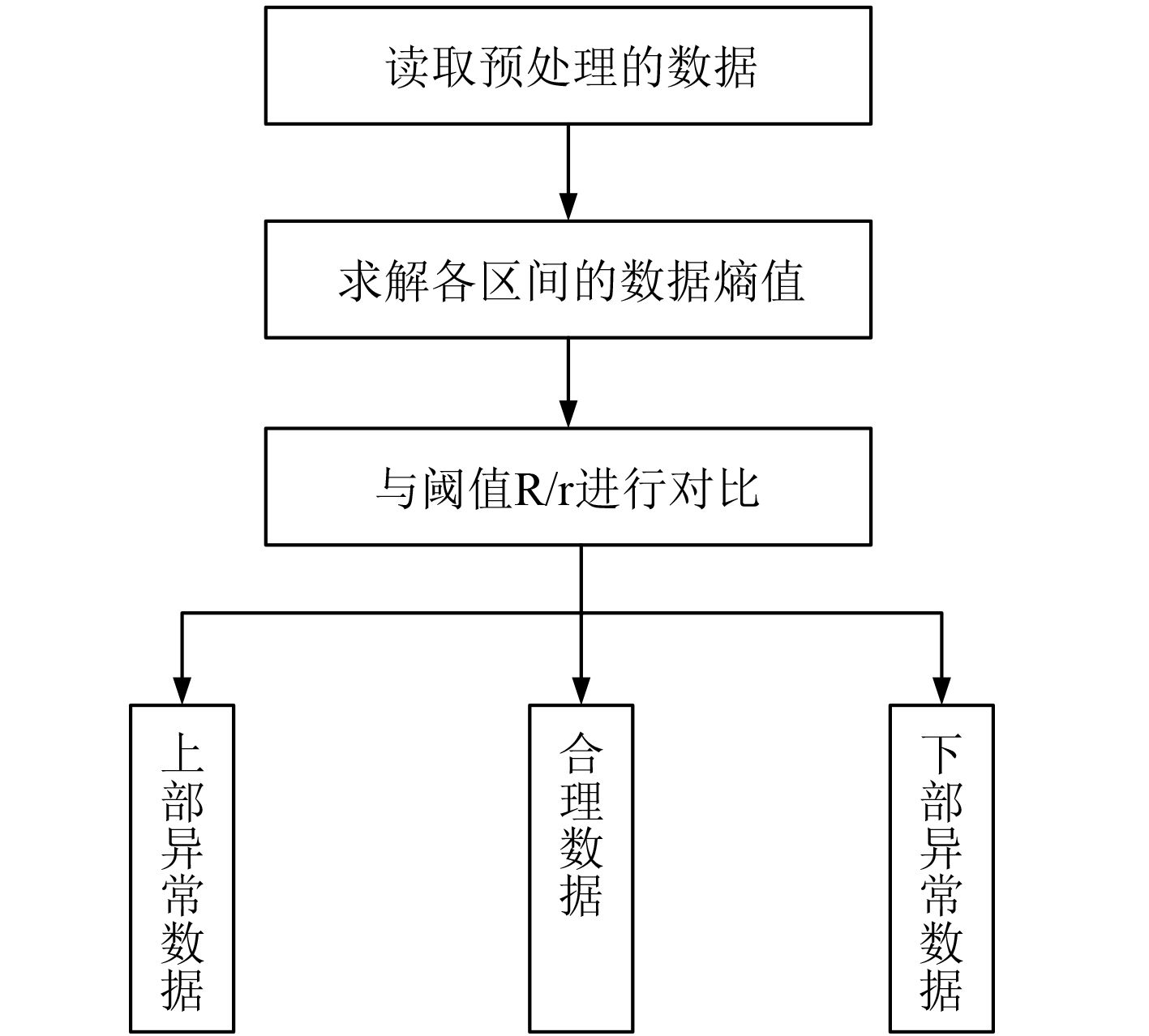图 1 基于云分段最优熵算法的异常数据识别流程 Fig. 1 Abnormal data identification process based on cloud segmentation optimal entropy algorithm

1）数据读取和预处理

 $E = \left( {E\left( 1 \right),E\left( 2 \right),\cdots,E\left( M \right)} \right) \text{。}$

2）熵值计算

3）数据分离

2 基于云分段最优熵算法的船舶航行异常轨迹识别技术 2.1 船舶航行异常轨迹识别系统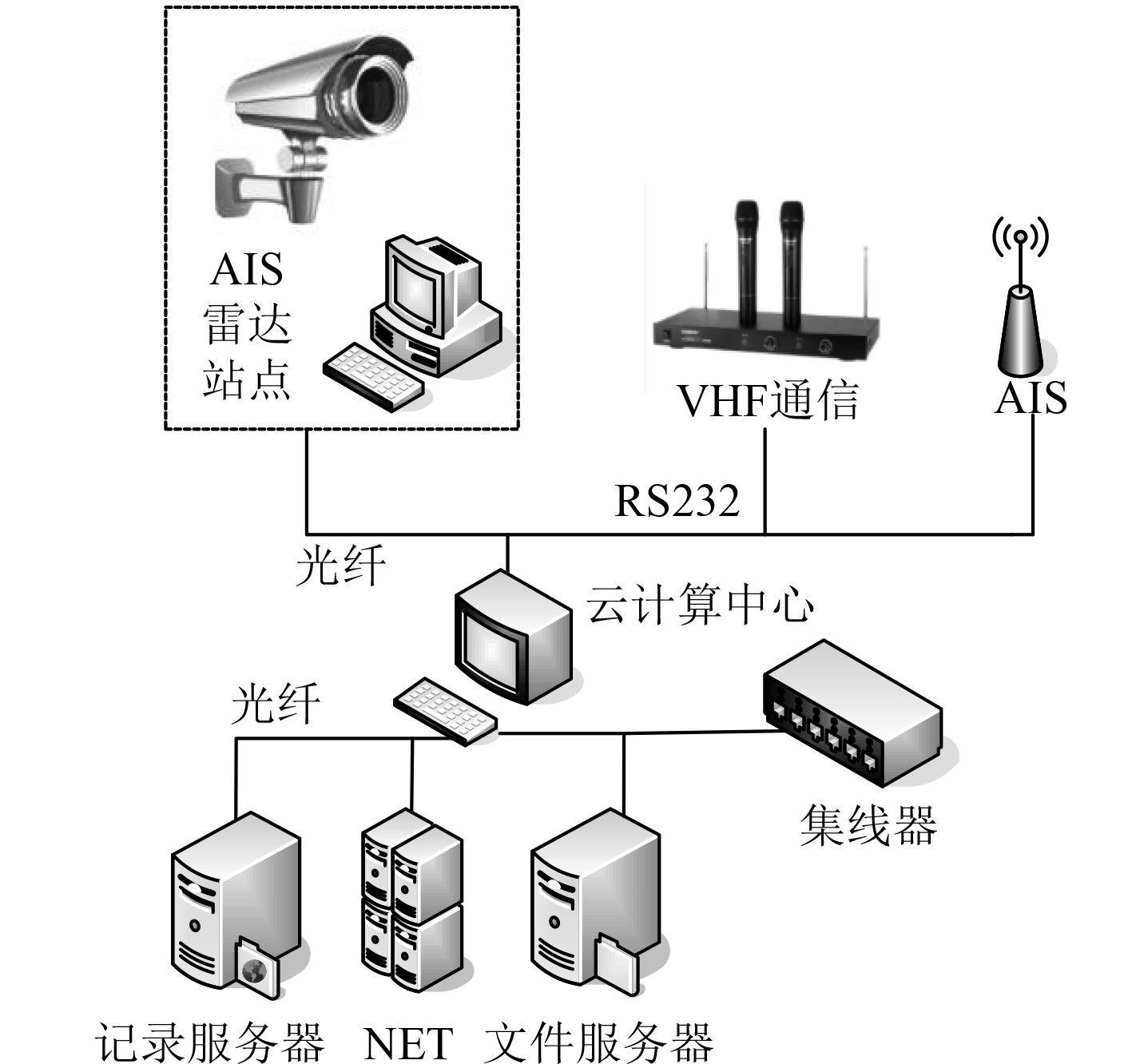图 2 船舶航行异常轨迹识别系统基本构成 Fig. 2 Basic structure of ship navigation abnormal trajectory recognition system

1）海量数据分析、转换和存储

2）航运异常数据识别

3）历史数据查询预测

2.2 船舶航行异常轨迹识别系统的轨迹监控功能设计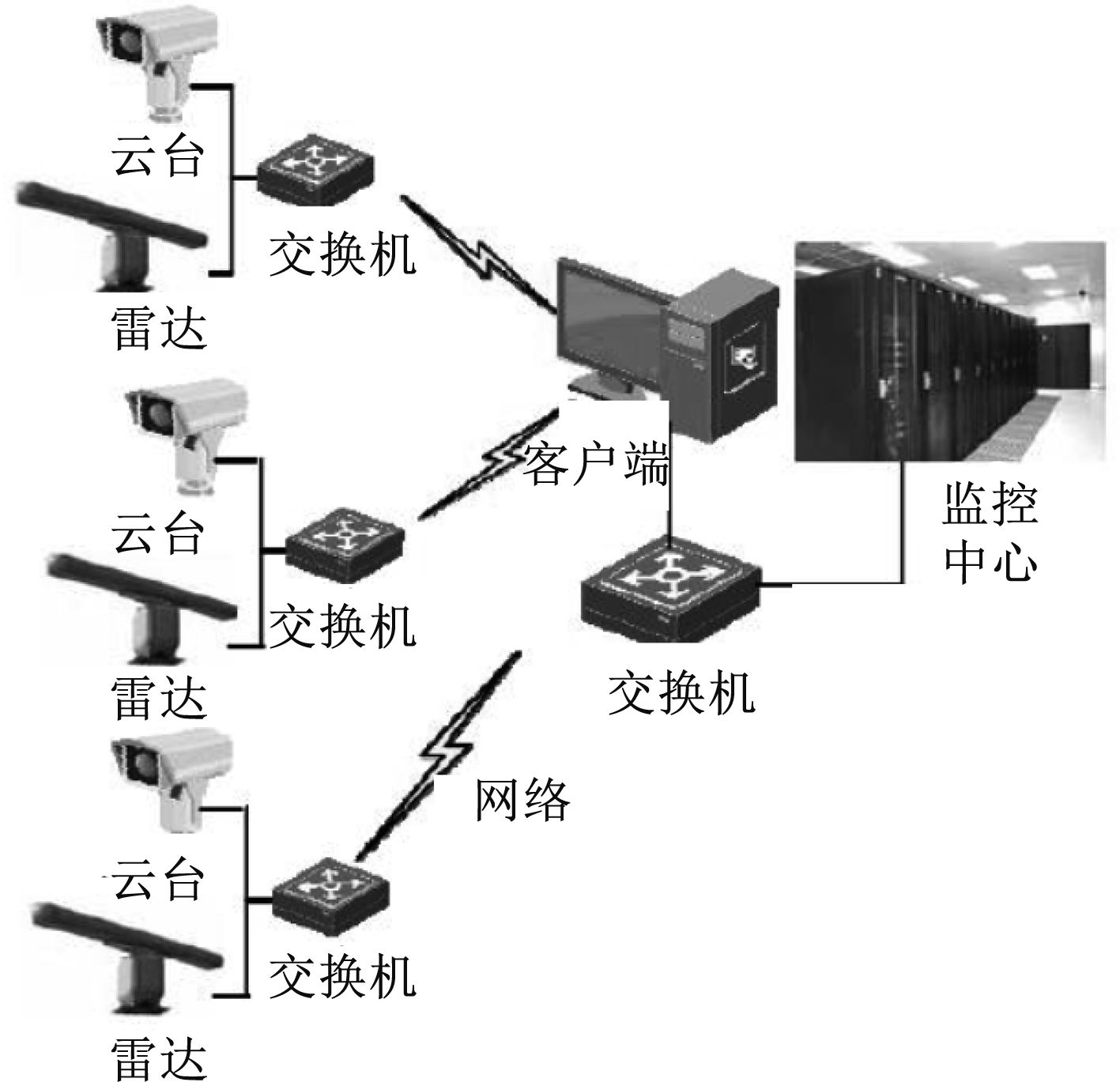图 3 视频监控模块的原理图 Fig. 3 Schematic diagram of video monitoring module

1）具备全天候监控和红外摄像功能，可实现24 h船舶的监控；

2）监控采集的图像质量清晰度高，分辨率为1 920×1 080；

3）具备船舶航运航迹的记录回放功能，每个雷达站的监控距离大于3 km；

4）客户端和监控中心可最高满足接入5路视频信号，并具备运算器和存储器的扩展功能。

2.3 船舶航迹数据压缩与挖掘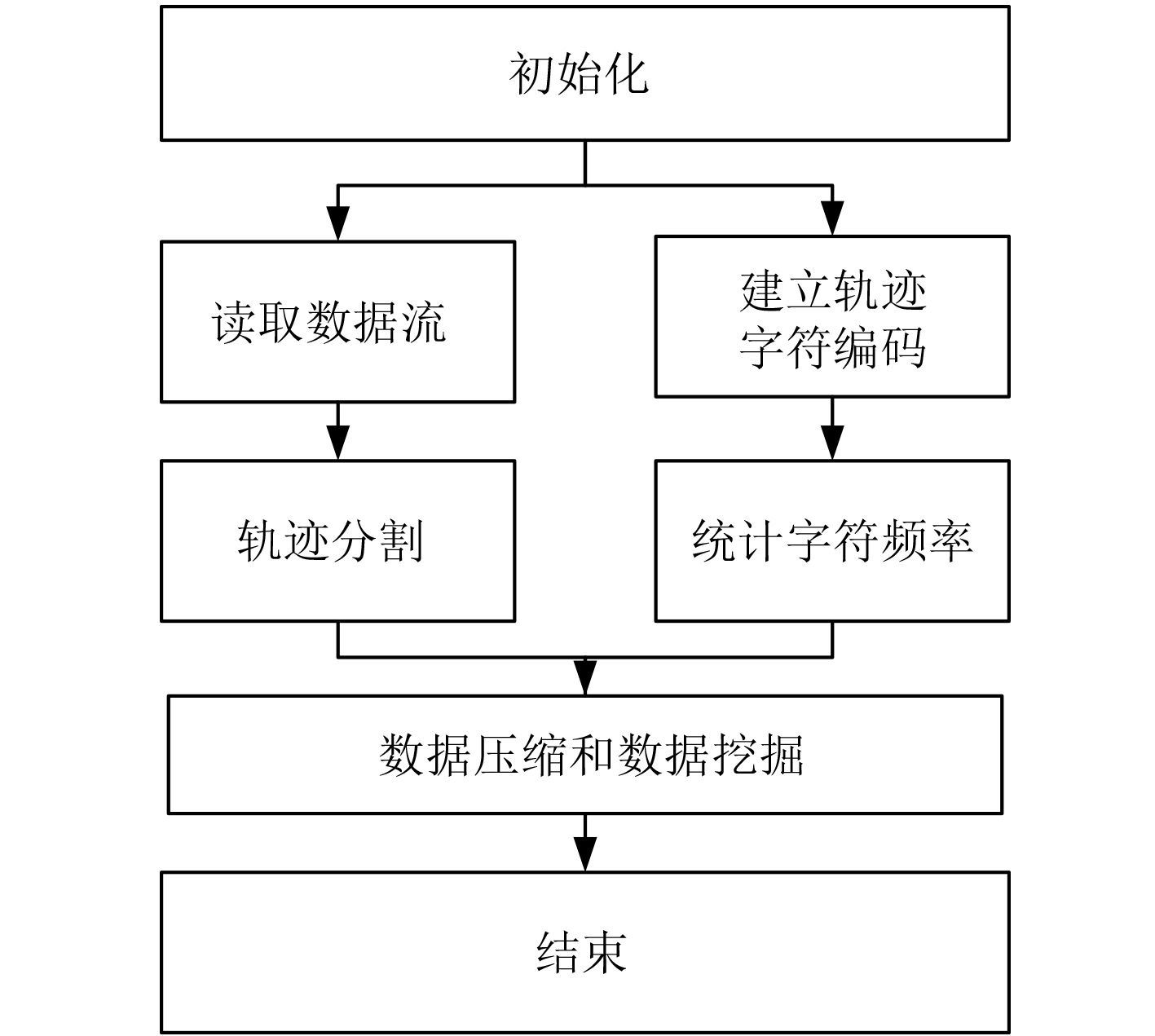图 4 基于船舶轨迹字符的数据压缩流程 Fig. 4 Data compression process based on ship track characters

 $C_R^{} = \frac{{{S_t}}}{{{S_r}}} \text{，}$

 $C_s^{} = 1 - C_R^{} 。$

 ${E_D} = \sum\limits_{i = 1}^n {\left| {dist\left( {{P_i} - {P_{i - 1}}} \right)} \right|} 。$
2.4 船舶航行异常轨迹数据识别表 1 云计算中心硬件参数表 Tab.1 Hardware parameter table of cloud computing center

1）采集一段时间的航迹数据，按照时间间隔0.05 s进行云分段，每个航迹特征点为 ${S_0}\left( {x,y} \right)$ ，航迹数据采集的滤波函数为：

 $\varphi (x,y) = \frac{1}{{{t^2} - 1}}\sum\limits_{s = 1}^s {f(x,y)} \text{，}$

2）将分段后的数据进行熵值计算，得到航迹数据的熵值曲线如图5所示。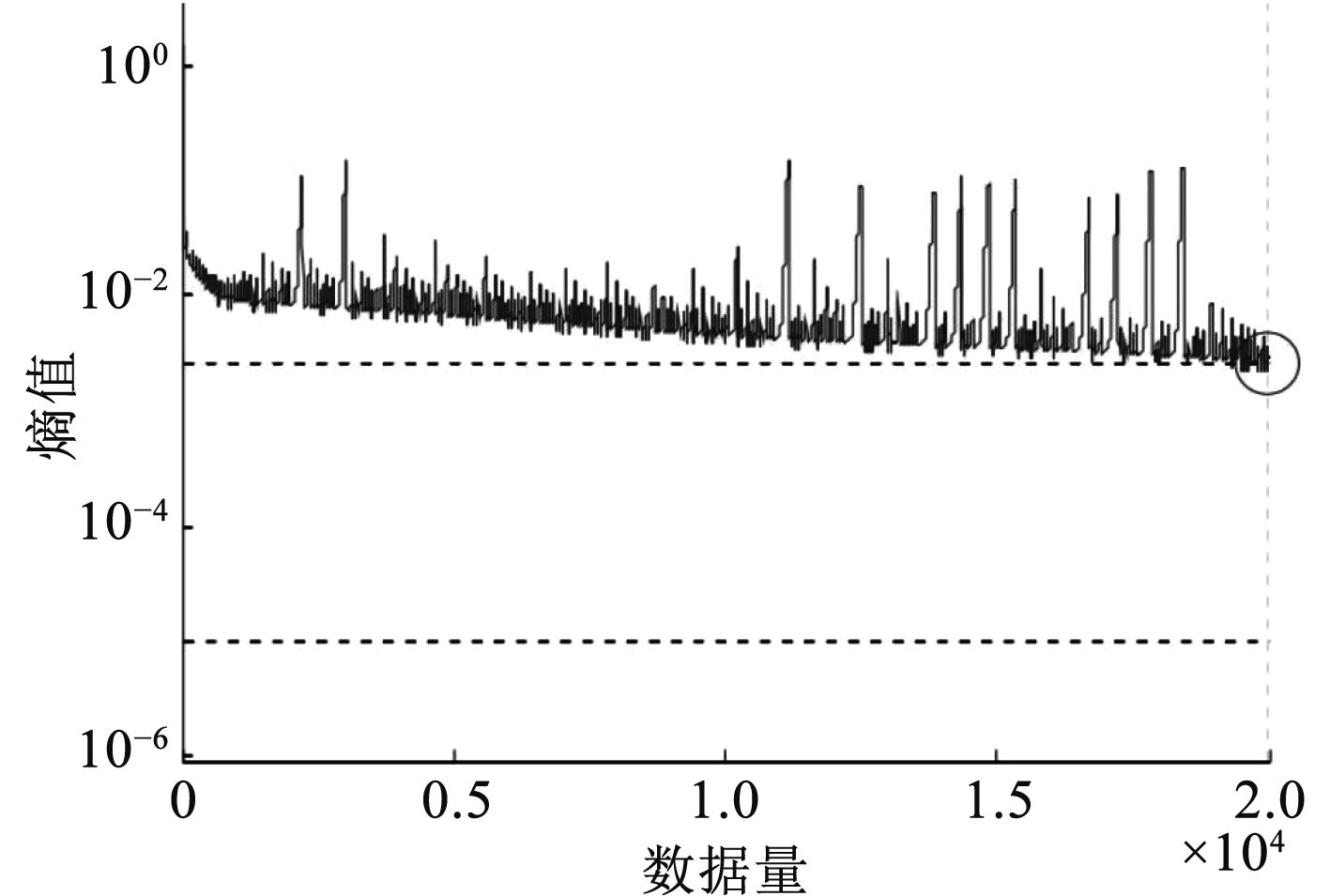图 5 船舶航迹数据的熵值曲线 Fig. 5 Entropy curve of ship track data

3）将船舶航迹数据的熵值与阈值R/r进行对比，获取船舶航行轨迹数据的异常数据识别结果，如图6所示。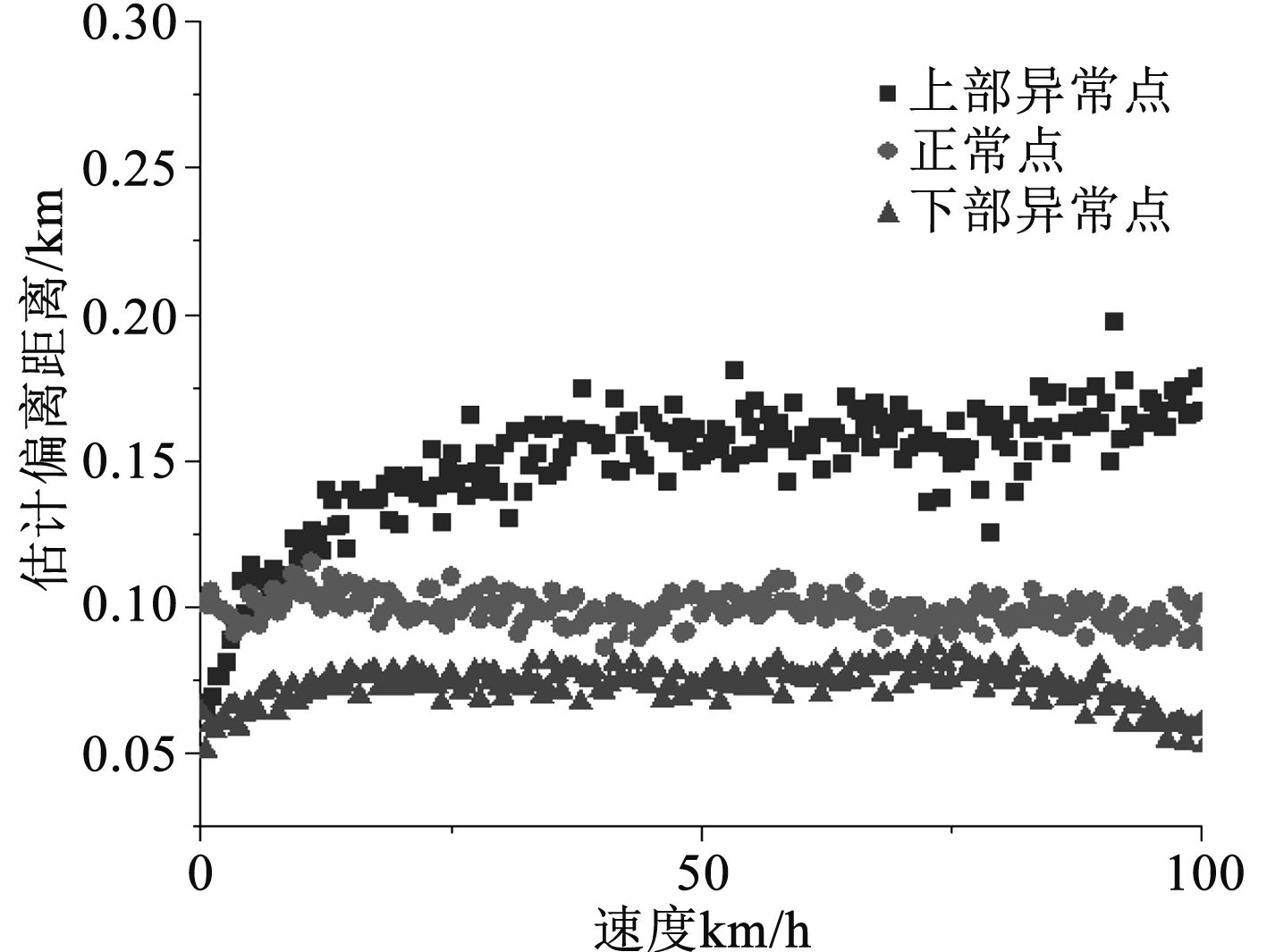图 6 船舶航行轨迹数据的异常数据识别结果 Fig. 6 Identification results of abnormal data of ship navigation trajectory data
3 结　语

  宋鑫, 朱宗良, 高银萍, 等. 动态阈值结合全局优化的船舶AIS轨迹在线压缩算法[J]. 计算机科学, 2019, 46(7): 6.  郑义成, 莫钦华, 王海鸿. 基于ElasticSearch的海量AIS数据存储方法[J]. 指挥信息系统与技术, 2016, 7(3): 6.  李名, 胡勤友, 孟良. 基于AIS的船舶运动轨迹压缩技术研究[J]. 航海技术, 2010(1): 3.  马瑞鑫, 杨凯, 尚东方. 基于船舶自动识别系统和高频地波雷达的船舶轨迹数据融合[J]. 2022(5).  姚高乐, 邓斌, 林泽强, 等. 船舶AIS数据处理系统的研究与实现[J]. 商品与质量, 2017(43): 10.